# MA2051 - Ordinary Differential EquationsSolutions to Sample Exam 2 - B96

### Second Exam - Originally Given 1995 D Term

1
A, z = -x,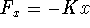B
;; M x'' = -Kx - Px'. With initial conditions, the final model is Mx'' + Px' + Kx = 0, x(0) = 0, x'(0) = 30.
C
The equation is linear and homogeneous.
D
Preventing oscillations requires real characteristic roots:. Hence, no oscillations if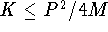; the spring can not be too strong lest the system oscillate.
E
Solve Mx'' + Kx = 0, x(0) = 0, x'(0) = 30 to find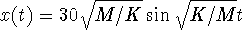. Maximum displacement (or amplitude) ofoccurs with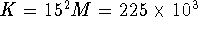N/m.

2
A
Via characteristic equation: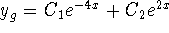B
Combine previous homogeneous solution with undetermined coefficients using: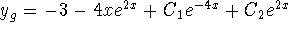C
Via characteristic equation: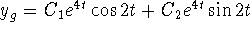D
Combine previous homogeneous solution with undetermined coefficients using :E
section 8.2, exercise 7
```

© 1996 by Will Brother.
All rights Reserved. File last modified on December 6, 1996.

```